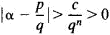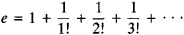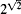# transcendental number

(redirected from Transcendentals)
Also found in: Dictionary, Thesaurus.

## transcendental number:

see numbernumber,
entity describing the magnitude or position of a mathematical object or extensions of these concepts. The Natural Numbers

Cardinal numbers describe the size of a collection of objects; two such collections have the same (cardinal) number of objects if their
.

## Transcendental Number

a real or imaginary number that is not algebraic. In other words, a transcendental number is a number that does not satisfy any algebraic equation with integer coefficients.

The existence of transcendental numbers was first established by J. Liouville in 1844. Liouville’s starting point was his theorem stating that the order of approximation of a given algebraic irrational number by a rational fraction with a given denominator cannot be made arbitrarily high. More precisely, if an algebraic number α satisfies an irreducible nth-degree algebraic equation with integer coefficients, then there exists a number c such thatfor any rational number p/q; here, c depends only on α. Therefore, the given irrational number α is transcendental if an infinite set of rational approximations can be found that do not satisfy the above inequality for any c and n (which are the same for all the approximations). An example of such a number isAnother proof of the existence of transcendental numbers was given by G. Cantor in 1874. He showed that the set of algebraic numbers is countable—that is, the algebraic numbers may be put into a one-to-one correspondence with the set of positive integers (seeSET THEORY). By contrast, the set of all real numbers is not countable. Consequently, the set of transcendental numbers is not countable; moreover, the transcendental numbers make up the bulk of the set of all numbers.

A fundamental problem in the theory of transcendental numbers is to determine whether the values of analytic functions with particular arithmetic and analytic properties are transcendental when the argument has algebraic values. Problems of this kind rank with the most difficult in modern mathematics. In 1873, C. Hermite proved that the base of the natural logarithmsis transcendental. The German mathematician F. Lindemann obtained in 1882 a more general result: if α is an algebraic number, then eα is a transcendental number. In 1930, Lindemann’s result was extended considerably by the German mathematician K. Siegel, who demonstrated, for example, the transcendence of the values of a broad class of cylindrical functions for algebraic values of the arguments.

At a mathematical congress in Paris in 1900, D. Hilbert listed 23 unsolved mathematical problems. One of them was whether the number αβ is transcendental if α and β are algebraic numbers and β is an irrational number. In particular, the question arises whether the numbersand eπ are transcendental. It may be noted that the problem of the transcendence of numbers of the type αβ was first stated in a special form by L. Euler in 1744. A complete solution of the problem was not obtained until 1934, when A. O. Gel’fond showed that such numbers are transcendental. From Gel’fond’s discovery it follows, in particular, that all common logarithms of natural numbers are transcendental.

Methods of the theory of transcendental numbers have been applied to a number of problems associated with the integer solution of equations.

### REFERENCE

Gel’fond, A. O. Transtsendentnye i algebraicheskie chisla. Moscow, 1952.

## transcendental number

[¦tran‚sen¦dent·əl ′nəm·bər]
(mathematics)
An irrational number that is the root of no polynomial with rational-number coefficients.
References in periodicals archive ?
Acceptance of the Lambert W function as a bona fide elementary transcendental function equal in importance to that of the well-established, traditional class of such functions, however, is expected to be no easy task.
The transcendentals, you see, have fallen on hard times.
In order to make the case for this understanding of the artist's creativity, I will first explain beauty as a "transcendental property of being" according to the Thomistic tradition as embodied by Maritain and Clarke.
(76) While Scotus maintains a certain logical place for common nature that might prima facie be misconstrued as another form of essentialism in the modern sense described earlier as a problem, he actually subordinates this as secondary to the more primary and intrinsic transcendental reality of haecceitas.
(2) Both thinkers show an aversion to abstract entities of various kinds, and attack one as a transcendental term, criticize the notion of privation, equate action with passion, and reject the additional moods of the first figure of the syllogism.
(42) In that way, Aquinas could benefit from Heidegger's development of phenomenological logic, which brings to intuitive givenness the transcendental relatedness of mind to world that Aquinas rightly considers.
For an analysis that argues that Aquinas did not recognize beauty as a transcendental, see Jan Aertsen, Medieval Philosophy and the Transcendentals: The Case of Thomas Aquinas (Leiden: E.
We might instead see it as tied up with what, in medieval thought, are called the transcendentals. The good is Being insofar as it is desired.
Added to these, and most objectionable, are mentioned in the following note reported to have been found on Kantor's nightstand at the time of his death: "No spirits, wraiths, hobgoblins, spooks, noumena, superstitions, transcendentals, mystics, invisible hands, supreme creator, angels, demons." (Kantor, 1984).
(2) Certainly his basic framework is the classical paradigm where all things participate by virtue of their existence in the essential Truth, Goodness, and Beauty of Being--a metaphysics inherited from Aquinas, Pseudo-Dionysius, and the Neoplatonists that holds fast to the transcendentals as the cascading outflow of the divine.

Site: Follow: Share:
Open / Close